Disclosure: This post may contain affiliate links, meaning when you click the links and make a purchase, we receive a commission.

# Excel formula to compare two columns and return a value (5 examples)

In this article, I will show you several techniques of using Excel formula to compare two columns and return a value. Your necessity might be different, so I will show several scenarios in my article.

Check out the scenarios and the relevant Excel formulas.

## 1) Compare two columns and return value from third column (using VLOOKUP formula)

In the following spreadsheet, you are seeing a list of some Projects and their Managers. In cell D2, the Project coordinator might input a Project name and want to see who the Manager of the Project is.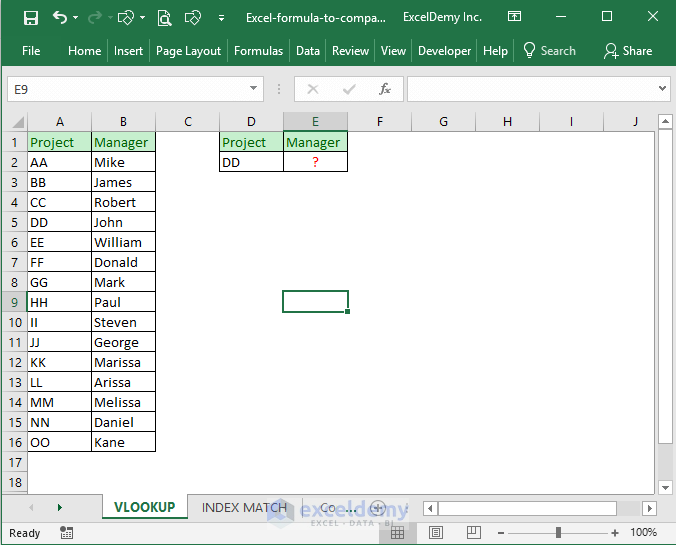Input this formula in cell `E2: =IFERROR(VLOOKUP(D2,project_manager,2,FALSE), "Not Assigned")`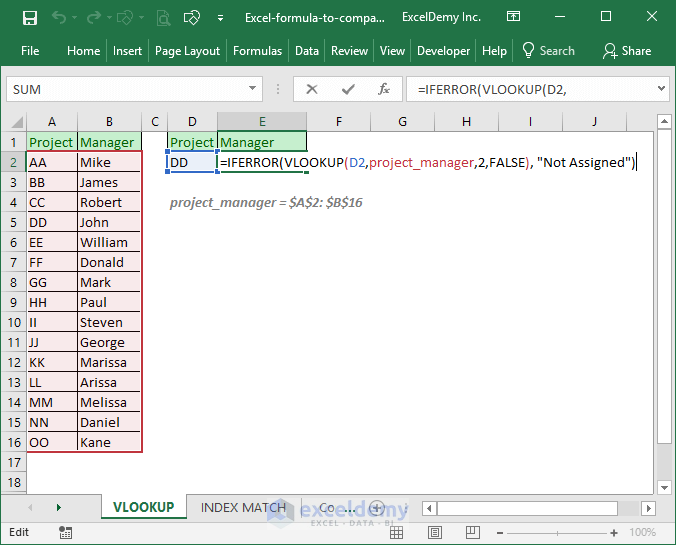### How does this formula work?

To understand this formula, you have to know the syntax of IFERROR and VLOOKUP Excel functions.

Take a look at the following image. If you observe the image carefully, you will find that the formula is actually self-explanatory.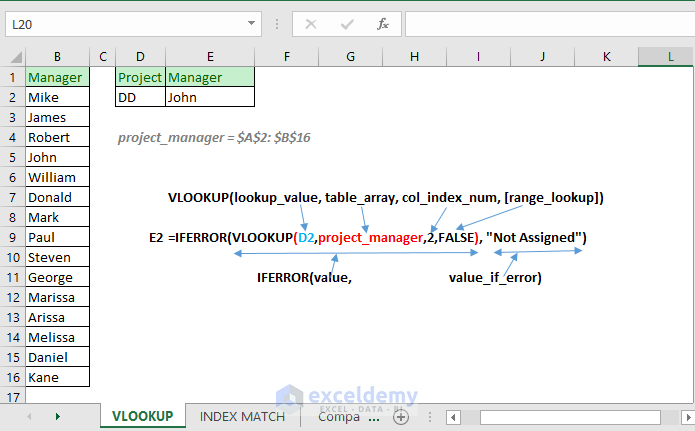If you don’t know how Excel’s VLOOKUP formula works, then give it a revision (from the image below)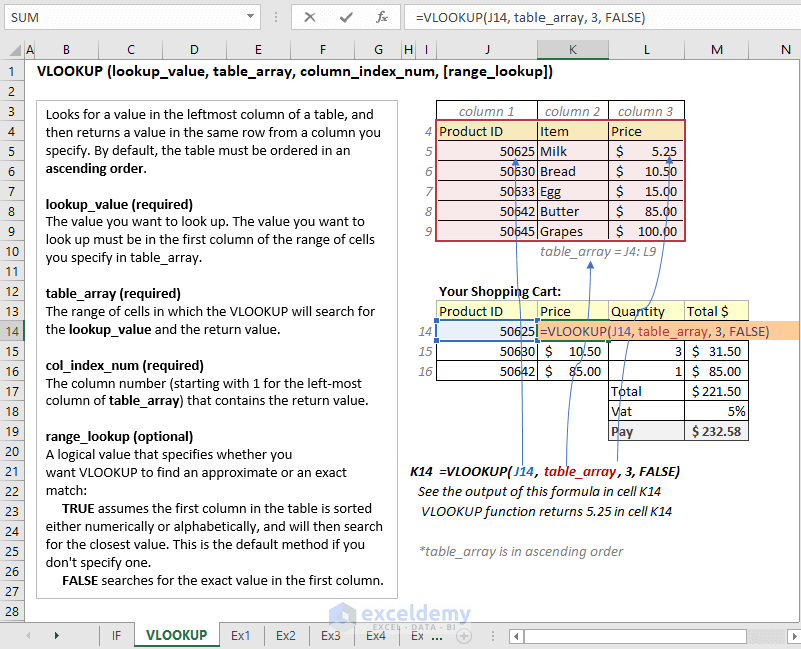## 2) Compare two columns and return value from the third column (using INDEX and MATCH functions)

We shall work again with the same data but this time we shall use this formula in cell `E2: =IFERROR(INDEX(B2:B16, MATCH(D2,A2:A16,0)), "")`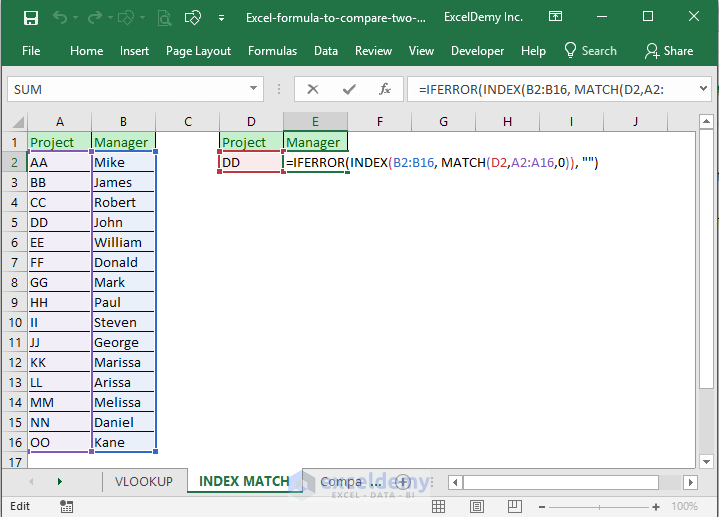This time if no matching is found, the cell will show blank as the IFERROR function’s value_if_error argument is blank.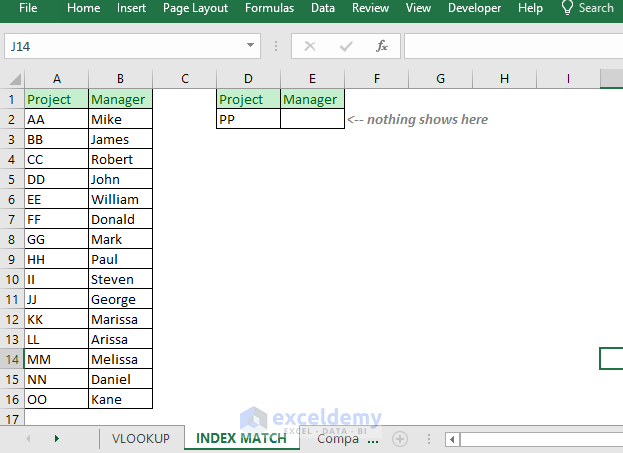### How does the formula work?

Check out the following image.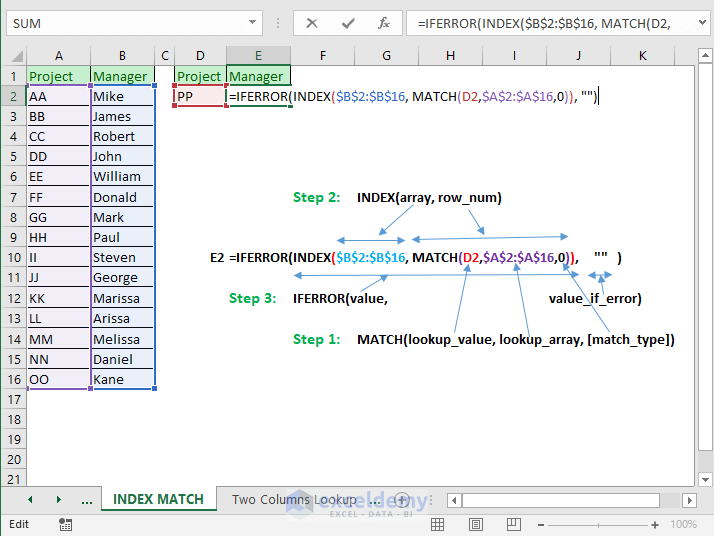These are the steps to analyze the above formula:

• Step 1: Match function returns the relative position of lookup_value D2. Say, the value is DD. It will return 4 as DD is in 4th position in the lookup_array \$A\$2:\$A\$16. If no matching was found, the MATCH function would return an error value.
• Step 2: INDEX function searches for the 4th value in the array \$B\$2:\$B\$16. And it finds value, John. So, for this example, the INDEX function will return value, John.
• Step 3: IFERROR function finds a valid value for its value So, it will return the value. If it would find an error value, then it would return a blank.

I hope you got how this formula works. Always try to break down a complex formula into pieces to make it easy.

## 3) Two columns lookup

This is another scenario. You have a worksheet like the following image.

You want to conduct a lookup on the table to match two different values from two columns. If matching is found, it will return data from the 3rd column.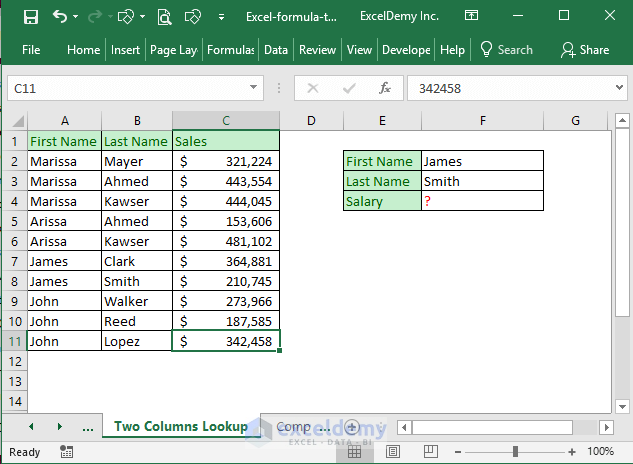You cannot perform two columns lookup with regular Excel formulas. You have to use an Array formula.

Input this formula in cell `F4: {=INDEX(C2:C11,MATCH(F2&F3, A2:A11&B2:B11,0))}`

At first, input the above formula in cell F4 without the curly braces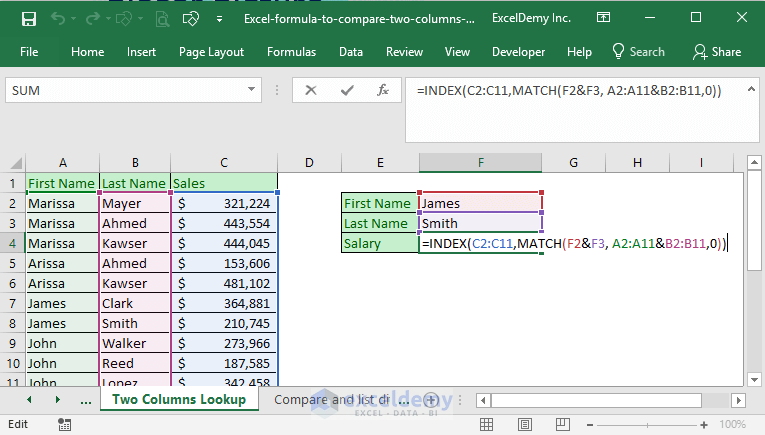Then press CTRL + SHIFT + ENTER on your keyboard. You will get the formula is input as an array formula.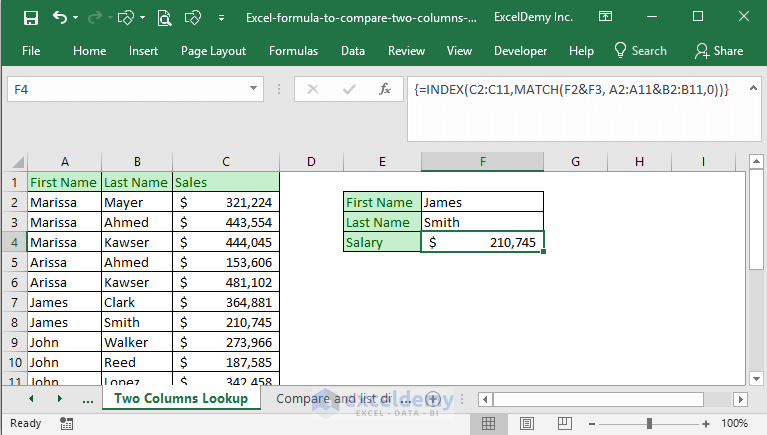### How does this array formula work?

At first, let’s understand the Match function part.

You can imagine this array formula as a series of the following formulas:

• MATCH(F2&F3, A2&B2,0)
• MATCH(F2&F3, A3&B3,0)
• MATCH(F2&F3, A4&B4,0)
• … … …
• … … …
• MATCH(F2&F3, A11&B11,0)

This series will be stored in Excel memory as `MATCH (JamesSmith, {"MarissaMayer"; "MarissaAhmed"; "MarissaKawser"; "ArissaAhmed"; "ArissaKawser"; "JamesClark"; "JamesSmith"; "JohnWalker"; "JohnReed"; "JohnLopez"}, 0)`

From the above MATCH function, what will be returned? MATCH function will return 7 as JamesSmith is found at position 7 of the array.

The rest is simple. In the array of C2:C11, the 7th position is value 210745. So, the overall function returns 210745 in cell F4.

If you fail to understand how this array formula works: check out my link.

## 4) Compare two columns and list differences in the third column

This is another scenario. You are seeing a worksheet below. There are two lists. What I want are:

• Compare the two lists
• Show the values of List 2 under a new column but without the values that are also in List 1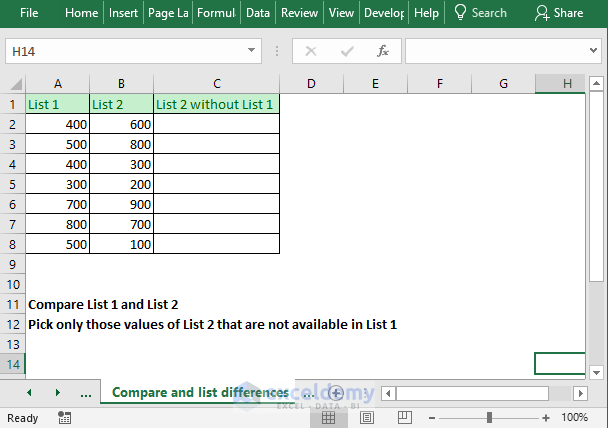We use this formula in cell `C2: =IF(ISNA(MATCH(B2, \$A\$2:\$A\$8,0)),B2, "")`

And then copy it for other cells in the column. We get the following results as shown in the figure below. You are seeing only those List 2 values are showing in the new column that is not in the List 1.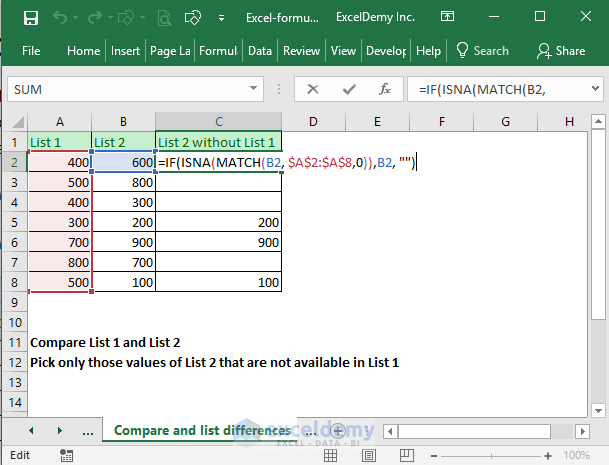### How does this formula work?

When you analyze a complex formula, start with the function that does not have any other functions in it. In this formula, that is the MATCH function.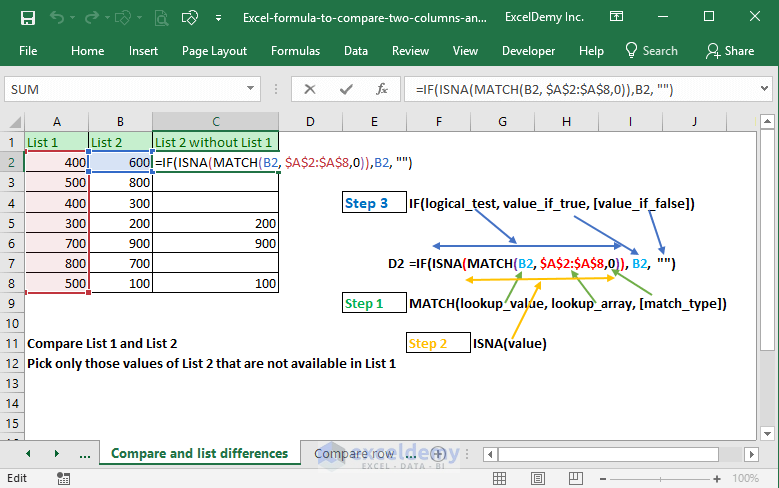Let’s break down the formula into pieces:

• Match function will search for cell value B2 in the range \$A\$2:\$A\$8. If it finds a match, it will return the position of the value, otherwise, it will return the #N/A. Value 600 of cell B2 is not found anywhere on the list. So, the Match function will return the #N/A error.
• ISNA function returns TRUE if it finds the #N/A error, otherwise, it will return a FALSE In this case, ISNA will return TRUE value as Match function returns #N/A error.
• When ISNA function returns a TRUE value, IF function’s value_if_true argument will be returned and it is B2, the value of B2 is 600. So, this formula will return 600 as value.

## 5) Compare two columns row by row

You might also want to compare two columns row by row like the following image.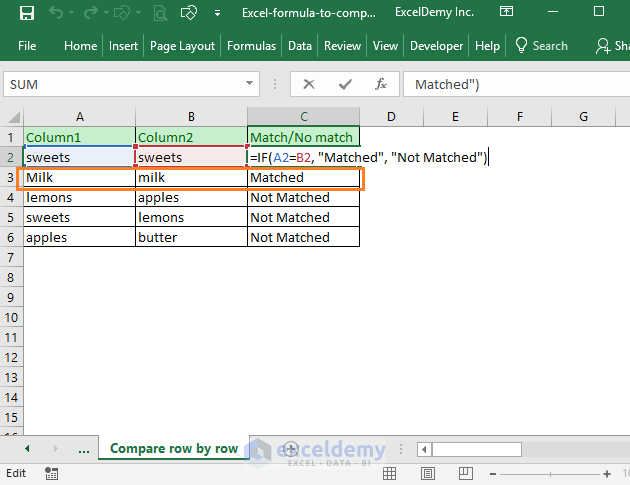We have used this formula in cell `C2: =IF(A2=B2, "Matched", "Not Matched")`

This is a straightforward Excel IF function. If the cells A2 and B2 are the same, “Matched” value will show in cell C2 and if the cells A2 and B2 are not the same, then the “Not Matched” value will show as the output.

This comparison is case-insensitive. “Milk” and “milk” are treated as same in this comparison.

We can use the EXACT function to find the exactly matched values. Just change the formula like this: `=IF(EXACT(A2,B2), "Matched", "Not Matched")`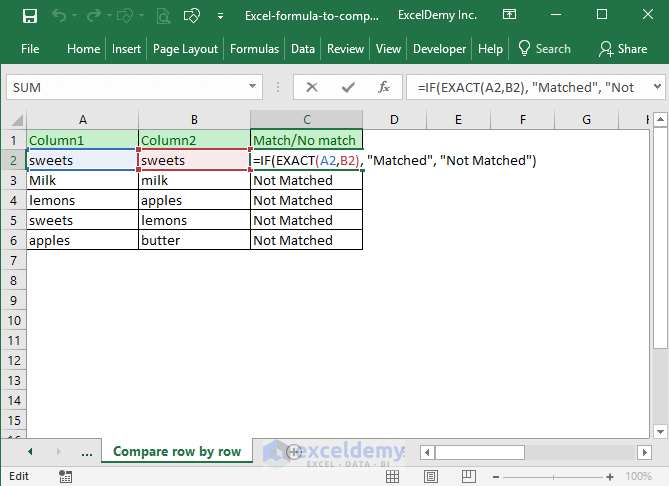You see now “Milk” and “milk” are treated differently. They are not the same.

Here is the syntax of the EXACT function. It takes two text values as arguments, if they are case-sensitively exact, the function returns the TRUE value, otherwise, the function returns the FALSE value.

EXACT (text1, text2)

So, these are my ways of comparing two columns in Excel and return a value based on the comparison. Do you know any other better techniques? Let me know in the comment box. I am eager to update this article.

Hello!
Welcome to my Excel blog! It took me some time to be a fan of Excel. But now I am a die-hard fan of MS Excel. I learn new ways of doing things with Excel and share here. Not only how to guide on Excel, but you will get also topics on Finance, Statistics, Data Analysis, and BI. Stay tuned!

1. Replymaier claudiu Jun 27, 2018 at 10:54 PM

Thank you very much. First of all, sorry for my bad english, than i want to congrats you for this lection and you have made in me one of the fan of your blog.

• Reply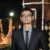Siam Hasan Khan Jun 28, 2018 at 12:32 PM

Thanks for your feedback. It means a lot to us. Keep following our website for more useful articles.

2. ReplyJOHN COUGHENOUR Jun 28, 2018 at 4:51 AM

Very clearly explained. This was a great help!

• ReplySiam Hasan Khan Jun 28, 2018 at 12:33 PM

Thanks for the feedback.

3. ReplyAhmad Akar Oct 23, 2018 at 7:09 PM

Thanks a lot, I liked your lesson, and liked your way of explaining (how does this formula work?) I like it so much. Thanks again.

4. ReplySurya May 2, 2019 at 3:25 PM

Thank you for this comprehensive and useful tutorial 🙂

5. ReplyRoger Whitacre Oct 10, 2019 at 1:10 AM

Thank you! Thank you! Thank you! Your formula: “3) Two Columns Lookup” solved a very complex problem for me. It also has automated a process that has been taking me quite a long time to do manually! Your examples made it very clear as to what I needed to do!

• ReplyOct 10, 2019 at 12:58 PM

Nice, Roger. I am glad to know that the formula helped you 🙂

6. ReplySunil Pinto Jun 12, 2020 at 12:44 AM

I have data in “A” column and input in “B” column and required result is in ” C” column.
I already found the result is in “E” Column. But this result needs to fix the corresponding raw of the A Column.

Data 1 Data 2 Required Result Formula
1 2 1 1
5 5 7
7 9 7 11
9 16 11
9 25
11 11 25
11 11 33
16 35
16 58
25 25 60
25 25 63
33 33 69

In E2 column array formula is :=IFERROR(IFERROR(INDEX(\$A\$2:\$A\$21, SMALL(IF(COUNTIF(\$B\$2:\$B\$21,\$A\$2:\$A\$21)=0, MATCH(ROW(\$A\$2:\$A\$21),ROW(\$A\$2:\$A\$21)), “”), ROWS(\$E\$2:E2))), INDEX(\$B\$2:\$B\$21, SMALL(IF(COUNTIF(\$A\$2:\$A\$21,\$B\$2:\$B\$21)=0, MATCH(ROW(\$B\$2:\$B\$21),ROW(\$B\$2:\$B\$21)), “”), ROWS(\$E\$2:E2)-SUM((COUNTIF(\$B\$2:\$B\$21, \$E\$2:\$E2)=0)+0)))),” “)